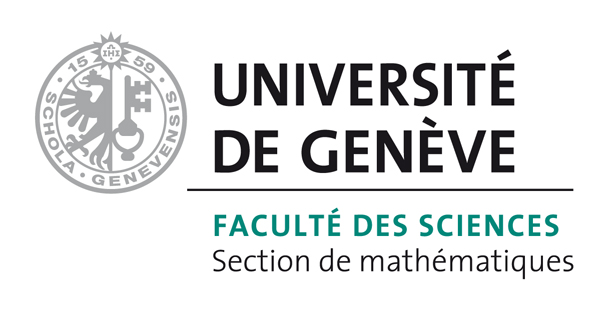# Bart Vandereycken## The geometry of algorithms using hierarchical tensors

by ,

#### Abstract:

In this paper, the differential geometry of the novel hierarchical Tucker format for tensors is derived. The set HT_k of tensors with fixed tree T and hierarchical rank k is shown to be a smooth quotient manifold, namely the set of orbits of a Lie group action corresponding to the non-unique basis representation of these hierarchical tensors. Explicit characterizations of the quotient manifold, its tangent space and the tangent space of HT_k are derived, suitable for high-dimensional problems. The usefulness of a complete geometric description is demonstrated by two typical applications. First, new convergence results for the nonlinear Gauss--Seidel method on HT_k are given. Notably and in contrast to earlier works on this subject, the task of minimizing the Rayleigh quotient is also addressed. Second, evolution equations for dynamic tensor approximation are formulated in terms of an explicit projection operator onto the tangent space of HT_k. In addition, a numerical comparison is made between this dynamical approach and the standard one based on truncated singular value decompositions.

#### Reference:

A. Uschmajew, B. Vandereycken, "The geometry of algorithms using hierarchical tensors", In Lin. Alg. Appl., vol. 439, pp. 133-166, 2013.

#### Bibtex Entry:

@article{Uschmajew_V_2013,
Abstract = {In this paper, the differential geometry of the novel hierarchical Tucker format for tensors is derived. The set HT_k of tensors with fixed tree T and hierarchical rank k is shown to be a smooth quotient manifold, namely the set of orbits of a Lie group action corresponding to the non-unique basis representation of these hierarchical tensors. Explicit characterizations of the quotient manifold, its tangent space and the tangent space of HT_k are derived, suitable for high-dimensional problems. The usefulness of a complete geometric description is demonstrated by two typical applications. First, new convergence results for the nonlinear Gauss--Seidel method on HT_k are given. Notably and in contrast to earlier works on this subject, the task of minimizing the Rayleigh quotient is also addressed. Second, evolution equations for dynamic tensor approximation are formulated in terms of an explicit projection operator onto the tangent space of HT_k. In addition, a numerical comparison is made between this dynamical approach and the standard one based on truncated singular value decompositions.},
Author = {Uschmajew, A. and Vandereycken, B.},
Doi = {10.1016/j.laa.2013.03.016},
Journal = {Lin. Alg. Appl.},
Pages = {133--166},
Pdf = {http://www.unige.ch/math/vandereycken/papers/preprint_Uschmajew_V_2013.pdf},
Title = {The geometry of algorithms using hierarchical tensors},
Volume = {439},
Year = {2013}}